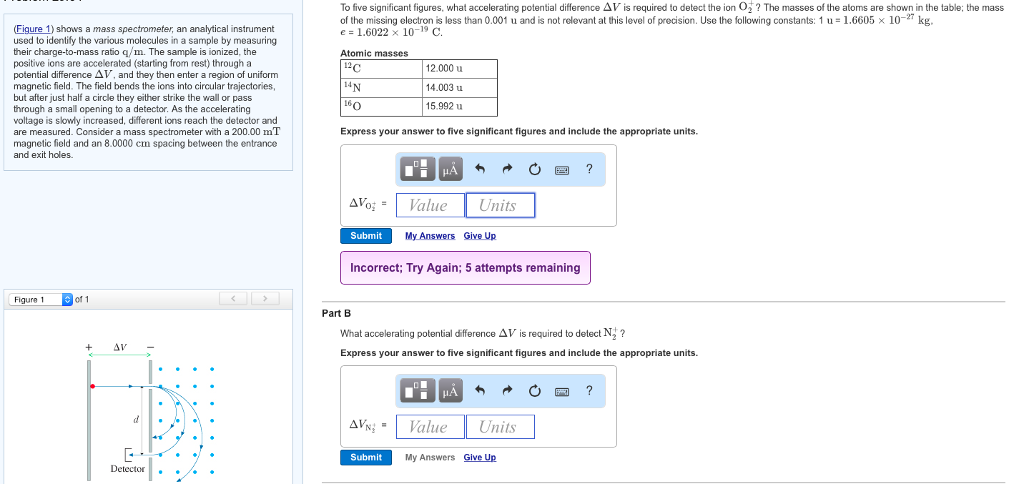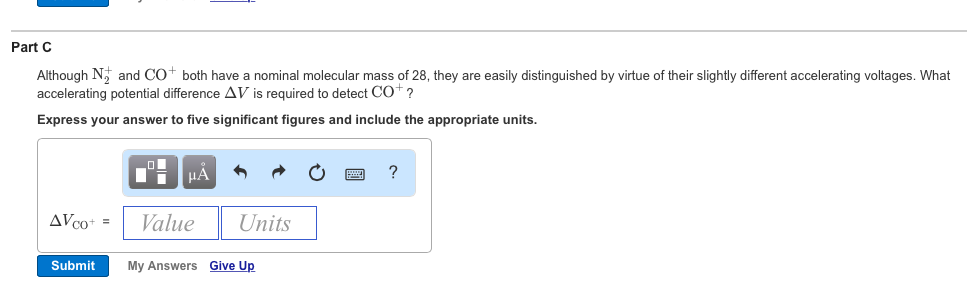# Question To five significant figures, what accelerating potential difference Δ V is required to detect the ion O: ? The masses of the atoms are shown in the table: the mass of the missing electron is less than 0.001 u and is not relevant at this level of precision. Use the following constants: 1 u = 1.6605 × 10-27 kg. e= 1.6022 x 10-19 C. Atomic masses Figure 1) To five significant figures, what accelerating potential difference Δ V is required to detect the ion O: ? The masses of the atoms are shown in the table: the mass of the missing electron is less than 0.001 u and is not relevant at this level of precision. Use the following constants: 1 u = 1.6605 × 10-27 kg. e= 1.6022 x 10-19 C. Atomic masses Figure 1) shows a mass spectrometer, an analytical instrument used to identify the various molecules in a sample by measuring their charge-to-mass ratio q/m. The sample is ionized, the positive ions potential difference ΔV, and they then enter a region of uniform magnetic field. The field bends the ions into circular trajectories but after just half a circle they either strike the wall or pass through a small opening to a detector. As the accelerating voltage is slowly increased, different ions reach the detector and are measured. Consider a mass spectrometer with a 200.00 mT magnetic field and an 8.0000 cm spacing between the entrance and exit holes are accelerated (starting from rest) through a 12 12.000 u 14.003 u 15.992 u L6 Express your answer to five significant figures and include the appropriate units. | Δ6_ Value Units Submit My Answers Give Up Incorrect; Try Again; 5 attempts remaining Figure 1 of 1 Part B What accelerating potential difference Δ V is required to detect N1 Express your answer to five significant figures and include the appropriate units. AVs:= | Value Units Submit My Answers Give Up Detector